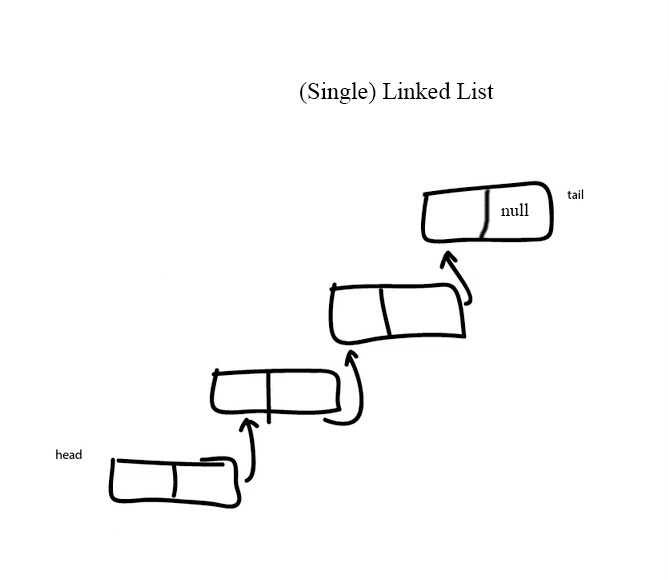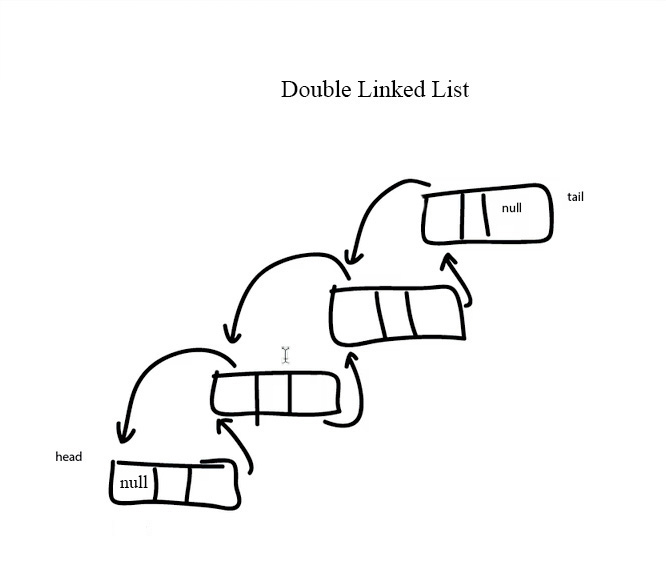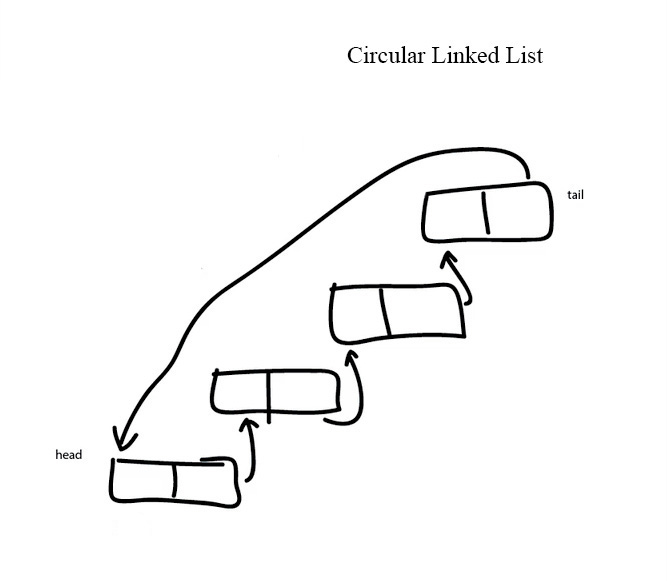Logout

5.1.13

Sketch linked lists (single, double and circular).

Teaching Note:

Students should be able to sketch diagrams illustrating: adding a data item to linked list, deleting specified data item, modifying the data held in the linked list, searching for a given data item.

Sample Question:

sdfsdfsf

JSR Notes:

***Note that there are a couple of minor errors with the videos, namely tail referred to as head in a couple of places. But the diagrams are corrected.

Sketching Basic Operations on Lists

(backup of video) (And there are a couple of little errors, can you spot them?)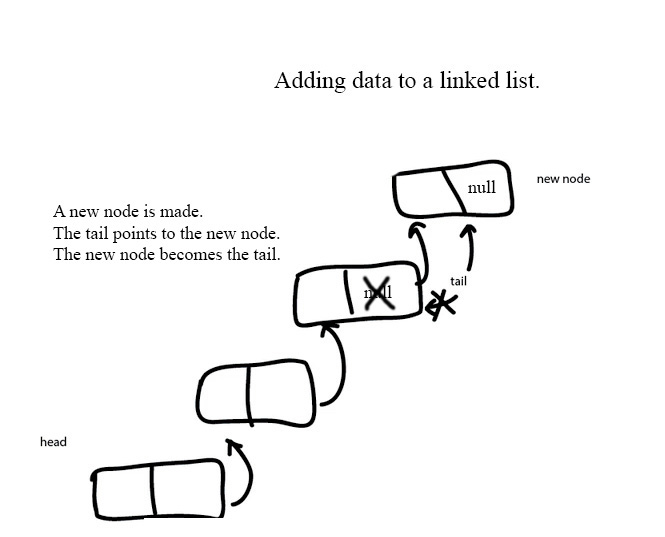And if you have seen and have a basic understanding of the code, the key line would be:
current.setNext(newNode);

Note above that with adding to a linked list, you can add to either end. Since this is the first time you are being shown this, I have picked adding to the end, which is arguably the easier of the two ways to understand, but not the most efficient.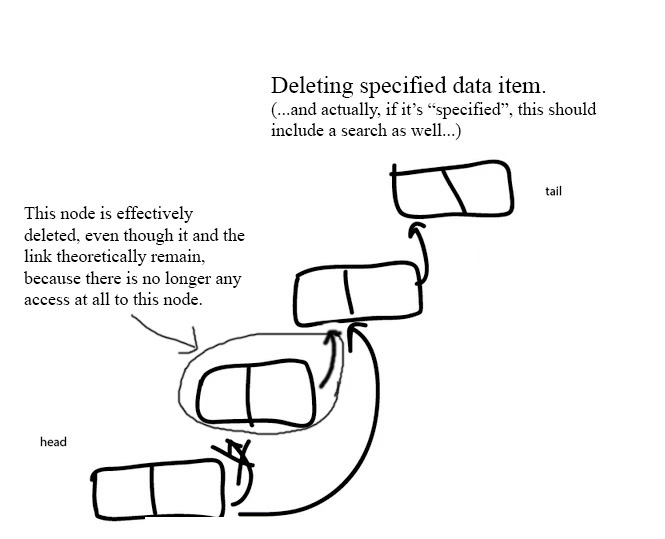And if you have seen and have a basic understanding of the code, the key line would be:
previous.setNext(current.getNext( ));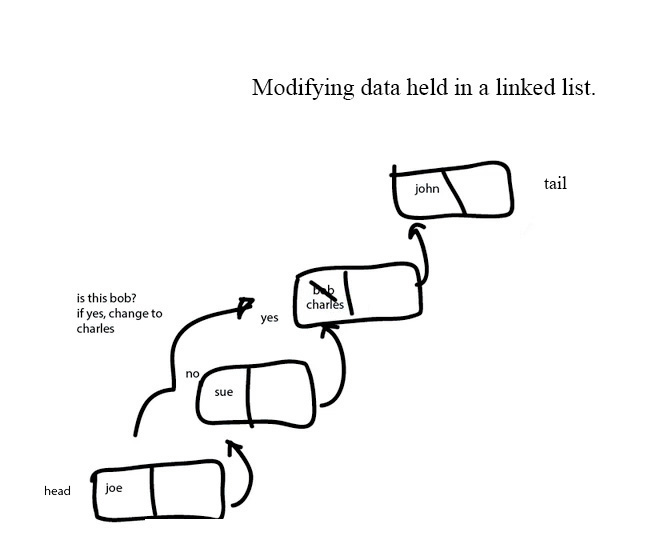And if you have seen and have a basic understanding of the code, the key line would be:
if(current.getData( ).getName( ).equals("bob")
current.getData( ).setName("charles");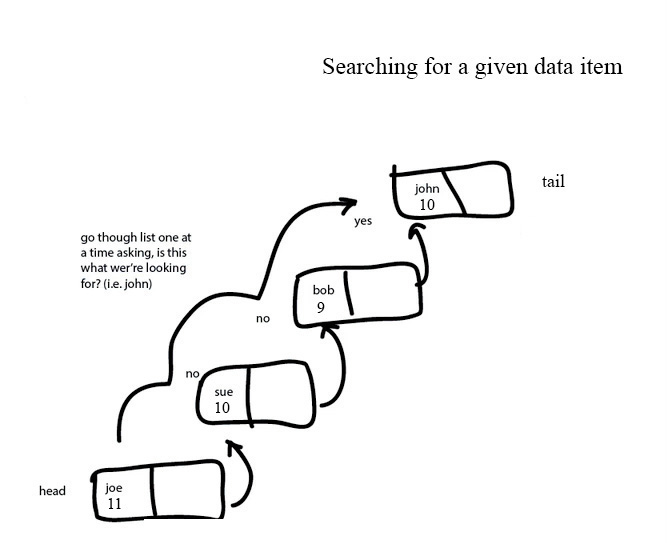And if you have seen and have a basic understanding of the code, a key line would be:
if(current.getData( ).getName( ).equals("bob")

Sketching Various Kinds of Lists